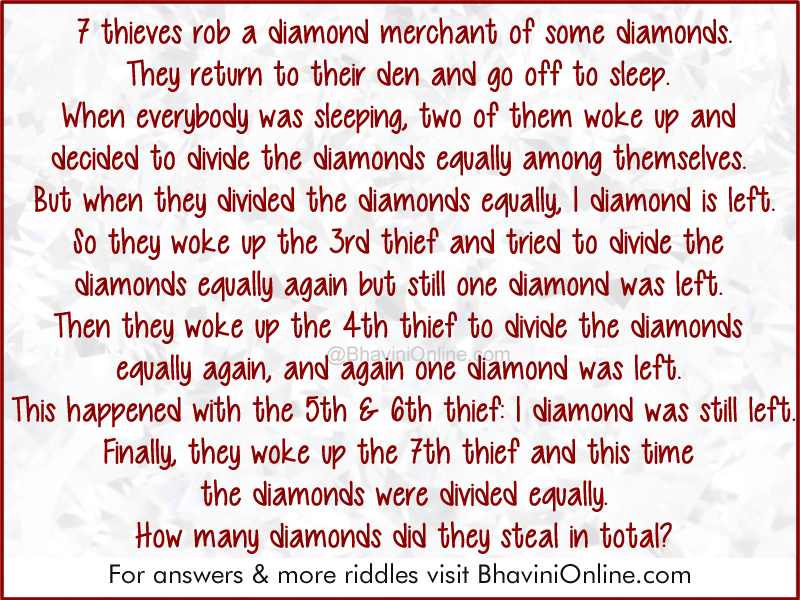# Logical Riddle: Equal Distribution of the Stolen Diamonds

Use your logical and mathematical skills to solve this riddle.

7 thieves rob a diamond merchant of some diamonds.

They return to their den and go off to sleep.

When everybody was sleeping, two of them woke up and decided to divide the diamonds equally among themselves.

But when they divided the diamonds equally, one diamond is left.

So they woke up the 3rd thief and tried to divide the diamonds equally again but still one diamond was left.

Then they woke up the 4th thief to divide the diamonds equally again, and again one diamond was left.

This happened with the 5th and 6th thief – one diamond was still left.

Finally, they woke up the 7th thief and this time the diamonds were divided equally.

How many diamonds did they steal in total?So were you able to solve the riddle? Leave your answers in the comment section below.

You can check if your answer is correct by clicking on show answer below. If you get the right answer, please do share the riddle with your friends and family on WhatsApp, Facebook and other social networking sites.

## 5 Replies to “Logical Riddle: Equal Distribution of the Stolen Diamonds”

1. samarth says:

they stole 7 diamonds

2. Sanjiv says:

Should be 49 as it looks 5th & 6th we’re woken together. Otherwise 301 is the right answer

3. MS PRIME says:

The thieves had 301 diamonds

4. There are multiple answers but I think 301 is the lowest and then 301 + 60*7 though I can’t quite figure out why that seems to work. 14 is even, so no go on 2. 21 is divisible by 3, 35 is the first number that would work for 2 and 3, then the first number where 4 also works would be 49, then the first number that 5 also works would be 301. (49*6+7). But that was just a brute force method. adding larger and larger sums. What’s the algebra/formula solution?

5. Suresh says:

721